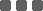## 3 Tips to make your code more pythonic

#### Programming

This article will introduce you to the top 3 ways you might not be fully taking advantage of to write better Python code, including:

1. List Comprehension
2. Dictionary Access
3. Lambda Function

# List Comprehension

A common programming task is to iterate the elements from an array and store the results in a new array. For example, you are given a list of numbers and want to transform it into a list of its squares. You may come up with something like this:

```arr = [1, 2, 3, 4, 5]

res = []
for i in arr:
res.append(i ** 2)

print(res)
```

Output:

```[1, 4, 9, 16, 25]

```

On the other hand, using the list comprehension approach, our codes can be simplified as follows:

```arr = [1, 2, 3, 4, 5]
res = [i ** 2 for i in arr]

print(res)
```

Output:

```[1, 4, 9, 16, 25]

```

If you are familiar with set notation in mathematics, list comprehensions may already look familiar. The usual steps to think about list comprehensions are as follows:

• ```[]
```

• The next thing that goes in the list comprehension is whatever you would put inside of the .append() using the for loop method
• ```[i ** 2]
```

• Finally put the for loop header at the end of the list
• ```[i ** 2 for i in arr]
```

# Dictionary Access

In Python, a dictionary is JSON data structure presented in a key-value pair. It allows fast value lookup based on a given key.

As wonderful as dictionaries are, our code may crash because of an unavailable key. This problem most often occurs when interfacing with external APIs because in many cases a key is available when some conditions met. To prevent this error, we may write:

```data = [
{"name":"Alice", "id":"100", "isStudent":True},
{"name":"Bob", "isStudent":False},
{"name":"Eva", "id":"105", "isStudent":True}
]

for d in data:
if "id" in d:
print(d["id"])
else:
print("Not a student!")
```

Output:

```100
Not a student!
105

```

However, a more pythonic way to write this is using dict.get(lookup_key, default_value)

```for d in data:
print(d.get("id","Not a student!"))
```

Output:

```100
Not a student!
105

```

# Lambda Function

In Python, lambda functions are those single-line, anonymous functions, which is useful when performing minor alterations to data. Lambda functions behave in the same way as regular def functions, but have the following characteristics:

• a lambda function can take any number of arguments, but they are restricted to only a single expression
• lambda functions can be used to return function objects

A lamda has the following syntax:

```lambda argument(s): expression

```

Example 1: Product of 2 numbers

```product = lambda x, y : x * y

print(product(8, 3))
```

In this code the lambda x, y: x*y is the lambda function. The x, y are the arguments while x * y is the expression that is evaluated and the result of the expression is returned. Running the code will output:

```24

```

The above lamda function is similar to

```def product(x, y):
return x*y
```

Example 2: Filter elements from a list

```arr = [1, 2, 3, 4, 5, 6, 7, 8, 9, 10]

res = list(filter(lambda i: (i < 6), arr))
print(res)
```

It will behave similar to below function:

```def fn_filter(arr):
res = []
for i in arr:
if i<6:
res.append(i)
return res
```

Output:

```[1, 2, 3, 4, 5]

```

Example 3: Squares of all items in a list

Here we will use the map() function which basically maps every item in the input iterable to the corresponding item in the output iterable, according to the logic defined by the lambda function. Consider the example to transform a list of numbers to its squares, apart from the list comprehension method above, we can also do it as follows:

```arr = [1, 2, 3, 4, 5]

res = list(map(lambda i: i**2, arr))
print(res)
```

Output:

```[1, 4, 9, 16, 25]

```

# Summary

Now you got it, our top 3 tips that will definitely help you writing better Python code in no time! If you find this article useful, don't forget to like and share with your friends!

Happy coding and have a good day!

Thanks for sharing~~

### Categories

Hot Topic

Is it a good time to invest now?

13 1 0 2022-09-25

3 Tips to make your code more pythonic

85 1 1 2022-09-16

Request for performance benchmark

111 2 0 2022-09-09

Example in Tutorials (Moving Average)

178 3 0 2022-09-07

Usage of Instruments Other than Crypto for Algo-Trading Crypto Challenge Competition

99 1 0 2022-09-06

Guideline to upload custom data via API

154 0 1 2022-09-05

How long should a backtest be?

161 5 0 2022-08-26

Connect Trading Account with WhaleFin

265 0 6 2022-08-18

No longer support trading services on Eqonex

129 1 0 2022-08-15

How to fetch market capitalization data

157 1 0 2022-08-15